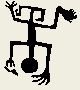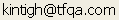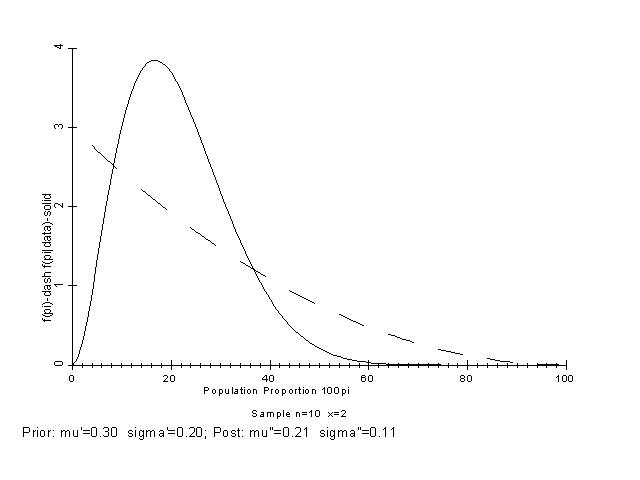Tools for Quantitative Archaeology    Email:BAYES: Bayesian Methods for Proportions

BAYES, a program still under development (and not currently supported) is included in the package for those interested. The program implements Bayesian methods for proportions as described by Iversen (1984). The notation used in prompts and in output follows Iversen. Intervals are calculated and graphed for Bayesian estimates of proportions based on both flat (uninformative) and informative priors. Informative priors can be described by their mean and standard deviation of a Beta distribution.Figure 12. Plot of Bayesian Prior and Posterior Probabilities.

PROGRAM EXAMPLE

```Output File Name {CON} ?
Create a Plot for a HPGL Plotter {N} ?

Bayesian Estimates for Proportions
[I]nformative or [F]lat Prior {F} ? I
Prior Beta Distribution µ { 0.000} ? .3
Prior Beta Distribution s { 0.000} ? .2
Sample n {0} ? 10
Sample x {0} ? 2

Sample n =  10  Sample x =   2
Prior a' =   1  Prior b' =   3  C' = 3.000E+00
Post a"  =   3  Post b"  =  11  C" = 8.580E+02
Prior µ' = 0.300  Prior s' = 0.200
Post µ"  = 0.214  Post s"  = 0.106

Print Bayesian Probability Intervals {Y} ? Y
Resolution of p (.001 - .01) {0.010} ?
Bayesian Probability Intervals
100%

Tail                         Interval
----------------  ------------------------------------------
Level     p0   P(p<=p0)     p1    p2   P(p1<=p<=p2)   f(p1)   f(p2)
0.01   0.040    0.014    0.170 0.180      0.038      3.847   3.821
0.05   0.070    0.058    0.160 0.180      0.077      3.842   3.821
0.10   0.090    0.105    0.150 0.180      0.115      3.801   3.821
0.20   0.130    0.234    0.140 0.200      0.228      3.722   3.685
0.25   0.140    0.270    0.130 0.200      0.265      3.602   3.685
0.50   0.210    0.535    0.100 0.240      0.503      2.992   3.177
0.75   0.280    0.751    0.070 0.310      0.761      2.035   2.017
0.80   0.310    0.819    0.060 0.330      0.816      1.664   1.703
0.90   0.360    0.900    0.040 0.380      0.910      0.913   1.040
0.95   0.420    0.957    0.030 0.430      0.957      0.569   0.574
0.99   0.510    0.991    0.000 0.990      1.000      0.000   0.000

Display Plot {Y} ?
Program End```

 Home Top Overview Ordering Documentation

Page Last Updated - 02-Jun-2007